Question

# A compound microscope consists of a 4× eyepiece & a 42× objective 30 cm apart. Assuming...

A compound microscope consists of a 4× eyepiece & a 42× objective 30 cm apart. Assuming the final image is in focus with the eye relaxed, determine the following:

Objective focal length =
Distance of object from objective =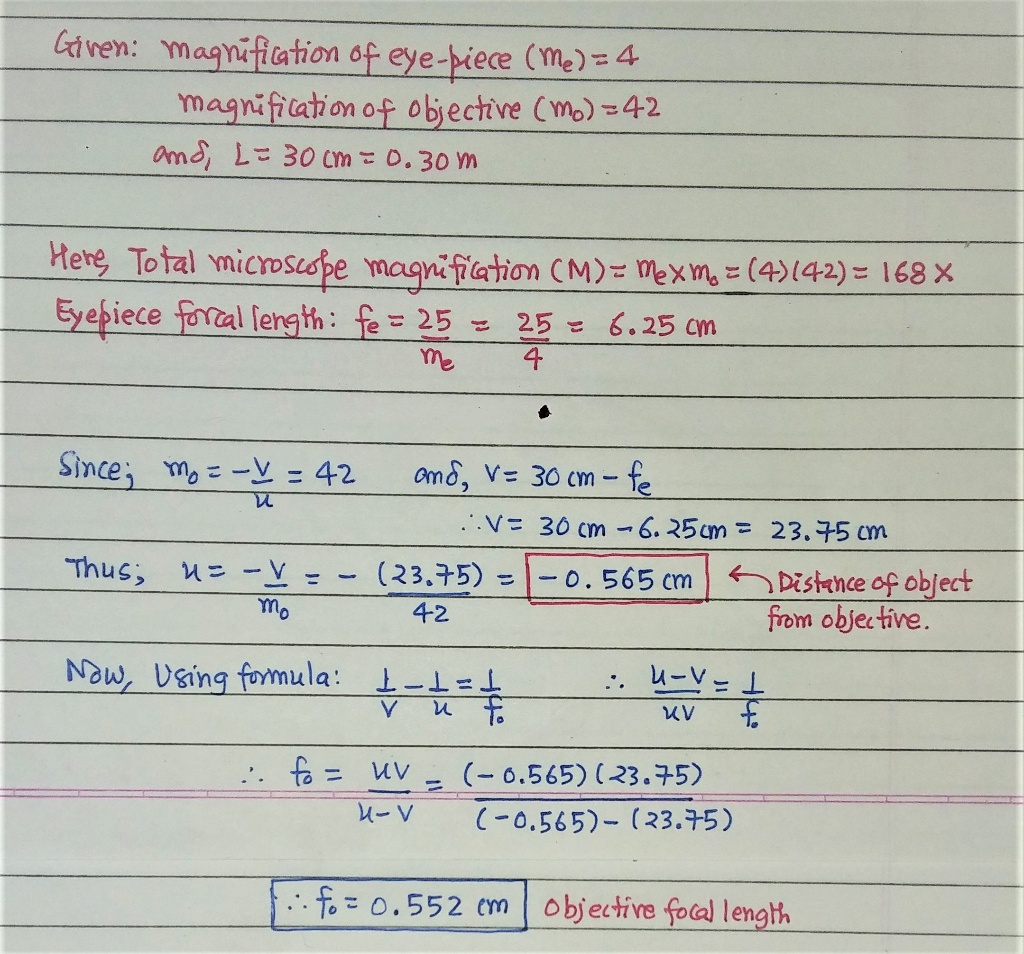Alright Dude, If that worked for you... don't forget to give THUMBS UP.(that will work for me!)
atleast before giving down vote.
and, Thanks for using Chegg- Smarter way to study.

#### Earn Coins

Coins can be redeemed for fabulous gifts.

Similar Homework Help Questions
• ### A compound microscope has the objective and eyepiece mounted in a tube that is 20.0 cm...

A compound microscope has the objective and eyepiece mounted in a tube that is 20.0 cm long. The focal length of the eyepiece is 2.72 cm , and the near-point distance of the person using the microscope is 25.0 cm. If the person can view the image produced by the microscope with a completely relaxed eye, and the magnification is -4025, what is the focal length of the objective?

• ### A microscope has a 9.0✕ eyepiece and a 63.0✕ objective 20.0 cm apart. (a) Calculate the...

A microscope has a 9.0✕ eyepiece and a 63.0✕ objective 20.0 cm apart. (a) Calculate the total magnification. ___________✕ (b) Calculate the focal length of each lens. eyepiece _________cm objective _________cm (c) Calculate where the object must be for a normal relaxed eye to see it in focus. __________cm

• ### 6. A compound microscope consists of two converging lenses (the objective and the eyepiece) placed 7.0...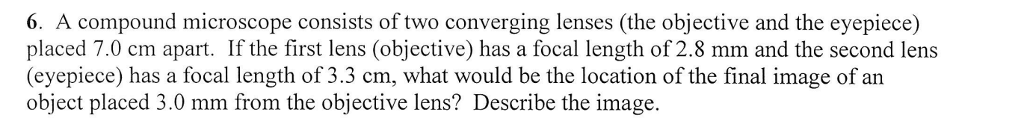6. A compound microscope consists of two converging lenses (the objective and the eyepiece) placed 7.0 cm apart. If the first lens (objective) has a focal length of 2.8 mm and the second lens (eyepiece) has a focal length of 3.3 cm, what would be the location of the final image of an object placed 3.0 mm from the objective lens? Describe the image

• ### The compound microscope consists of two converging lenses: the objective and the eyepiece. Suppose the focal...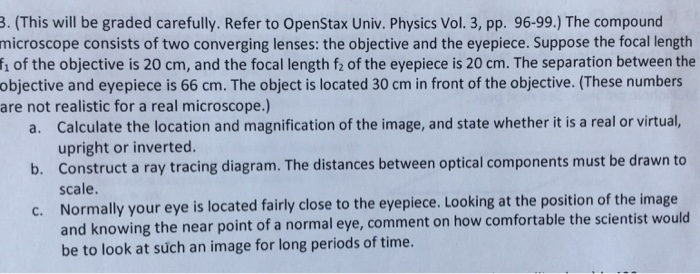The compound microscope consists of two converging lenses: the objective and the eyepiece. Suppose the focal length f_1 of the objective is 20 cm, and the focal length f2 of the eyepiece is 20 cm. The separation between the objective and eyepiece is 66 cm. The object is located 30 cm in front of the objective. (These numbers are not realistic for a real microscope.) a. Calculate the location and magnification of the image, and state whether it is a...

• ### an object is 1.2 cm form the objective lens of a simple compound microscope that is...

an object is 1.2 cm form the objective lens of a simple compound microscope that is adjusted for a relaxed normal eye. the objective and eyepiece are 18 cm apart and the eyepiece has a focal length of 4.8 cm. what is the focal length of the objective lens?

• ### Consider a microscope that consists of an objective lens and an eyepiece separated by 19.7 cm....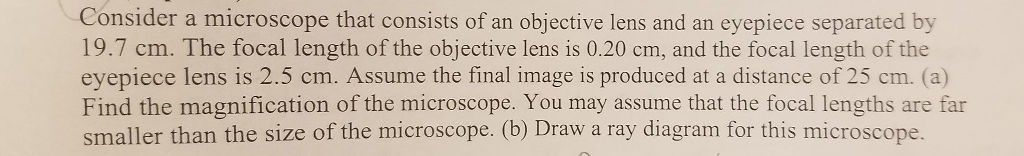Consider a microscope that consists of an objective lens and an eyepiece separated by 19.7 cm. The focal length of the objective lens is 0.20 cm, and the focal length of the eyepiece lens is 2.5 cm. Assume the final image is produced at a distance of 25 cm. (a) Find the magnification of the microscope. You may assume that the focal lengths are far smaller than the size of the microscope. (b) Draw a ray diagram for this microscope.

• ### Microscope - New A microscope has a 13.0 x eyepiece and a 64.0 x objective lens...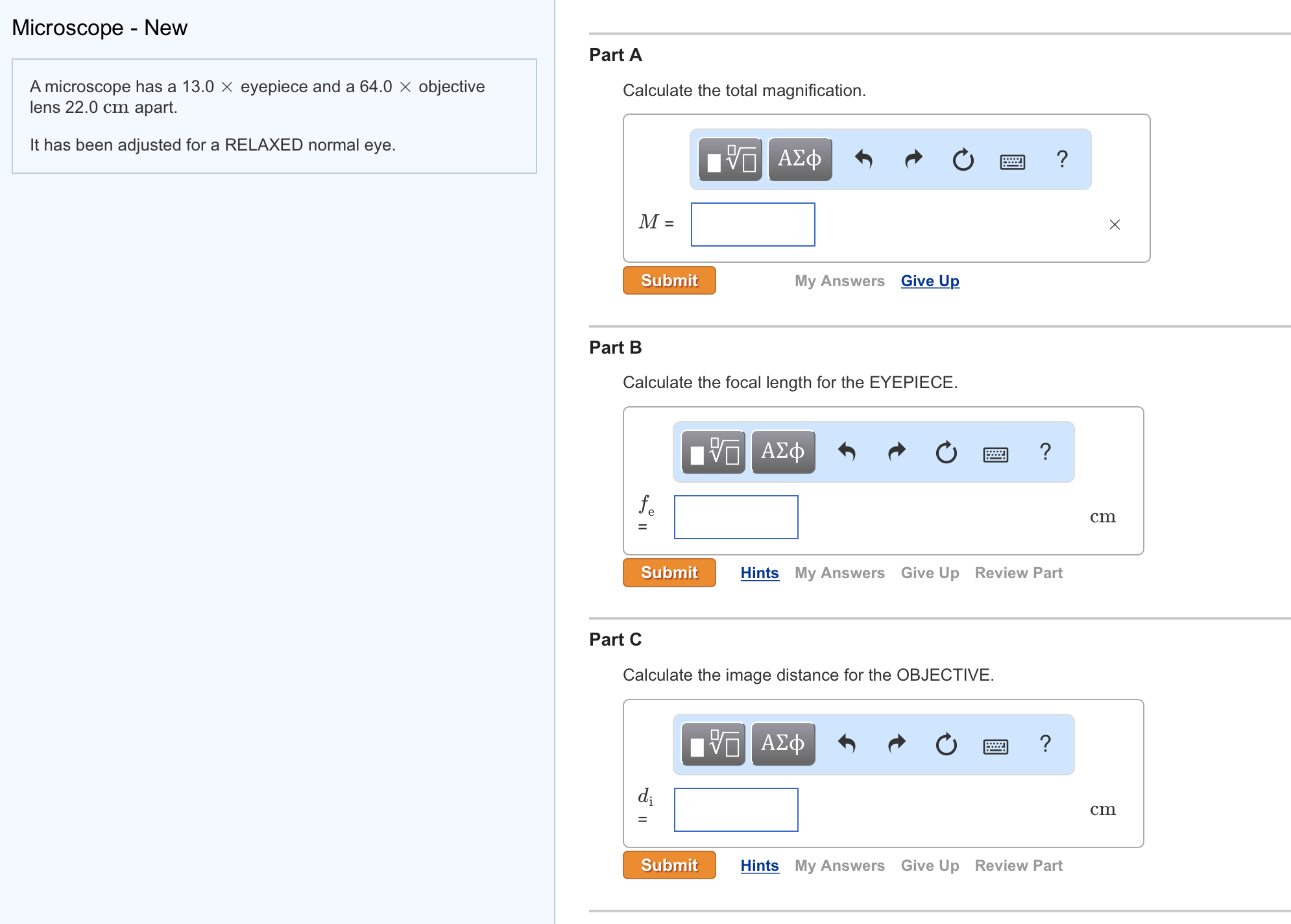Microscope - New A microscope has a 13.0 x eyepiece and a 64.0 x objective lens 22.0 cm apart. It has been adjusted for a RELAXED normal eye. Part A Calculate the total magnification. Part B Calculate the focal length for the EYEPIECE. Part C Calculate the image distance for the OBJECTIVE.

• ### The objective lens and the eyepiece of a microscope are 16.5 cm apart. The objective lens...

The objective lens and the eyepiece of a microscope are 16.5 cm apart. The objective lens has a magnification of 61 × and the eyepiece has a magnification of 11 ×. Assume that the image of the objective lies very close to the focal point of the eyepiece. A) Calculate the overall magnification of the microscope. B) Calculate the focal length of the eyepiece. C) Calculate the focal length of the objective.

• ### The microscope available in your biology lab has a converging lens (the eyepiece) with a focal length of 2.50 cm mounted...

The microscope available in your biology lab has a converging lens (the eyepiece) with a focal length of 2.50 cm mounted on one end of a tube of adjustable length. At the other end is another converging lens (the objective) that has a focal length of 1.00 cm. When you place the sample to be examined at a distance of 1.30 cm from the objective, at what length will you need to adjust the tube of the microscope in order...

• ### The focal lengths of the objective and the eyepiece in a microscope are 0.25 cm and...

The focal lengths of the objective and the eyepiece in a microscope are 0.25 cm and 1.0 cm, respectively. An object is placed at 0.30 cm from the objective lens and the image of this object is viewed with the eyepiece adjusted for minimum eyestrain. The near-point distance of the person using the microscope is 25.0 cm. What is the final magnification of the microscope?